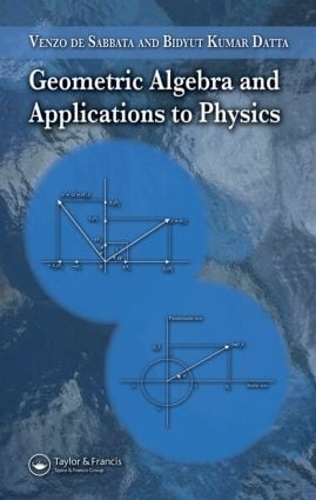•# Geometric Algebra and Applications to Physics (Hardback)

(author), (author)
£100.00
Hardback 184 Pages / Published: 07/12/2006
• We can order this

Usually dispatched within 3 weeks

Bringing geometric algebra to the mainstream of physics pedagogy, Geometric Algebra and Applications to Physics not only presents geometric algebra as a discipline within mathematical physics, but the book also shows how geometric algebra can be applied to numerous fundamental problems in physics, especially in experimental situations.

This reference begins with several chapters that present the mathematical fundamentals of geometric algebra. It introduces the essential features of postulates and their underlying framework; bivectors, multivectors, and their operators; spinor and Lorentz rotations; and Clifford algebra. The book also extends some of these topics into three dimensions. Subsequent chapters apply these fundamentals to various common physical scenarios. The authors show how Maxwell's equations can be expressed and manipulated via space-time algebra and how geometric algebra reveals electromagnetic waves' states of polarization. In addition, they connect geometric algebra and quantum theory, discussing the Dirac equation, wave functions, and fiber bundles. The final chapter focuses on the application of geometric algebra to problems of the quantization of gravity.

By covering the powerful methodology of applying geometric algebra to all branches of physics, this book provides a pioneering text for undergraduate and graduate students as well as a useful reference for researchers in the field.

Publisher: Taylor & Francis Inc
ISBN: 9781584887720
Number of pages: 184
Weight: 408 g
Dimensions: 235 x 159 x 15 mm